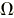# Physics: SI units and commonly used derived units

Syllabus: PC_BK_07, PC_BK_08

This page covers commonly used SI units and derived units, an exam favourite!

##### Definitions

The acronym for the SI units is SMMACKK –

Second, meter, mole, ampere, candela, kilogram and kelvin.

##### Second – time

The second (s) is defined by taking the fixed numerical value of the cesium frequency ∆νCs, the unperturbed ground-state hyperfine transition frequency of the cesium-133 atom, to be 9,192,631,770 when expressed in the unit Hz, which is equal to s−1.

##### Meter – distance

Meter: The meter (m) is defined by taking the fixed numerical value of the speed of light in vacuum c to be 299,792,458 when expressed in the unit m s−1, where the second is defined in terms of ∆νCs.

##### Mole – amount of substance

One mole (mol) contains exactly 6.02214076 × 1023 elementary entities. This number is the fixed numerical value of the Avogadro constant, NA, when expressed in the unit mol−1 and is called the Avogadro number. The amount of substance, symbol n, of a system is a measure of the number of specified elementary entities. An elementary entity may be an atom, a molecule, an ion, an electron, any other particle or specified group of particles.

When the mole is used, the elementary entities must be specified and may be atoms, molecules, ions, electrons, other particles, or specified groups of such particles.

##### Ampere – current

The ampere is defined by taking the fixed numerical value of the elementary charge e to be 1.602176634 × 10−19 when expressed in the unit C, which is equal to A s, where the second is defined in terms of ∆νCs.

##### Candela – luminous intensity

The candela (cd) is defined by taking the fixed numerical value of the luminous efficacy of monochromatic radiation of frequency 540 × 1012 Hz, Kcd, to be 683 when expressed in the unit lm

##### Kilogram – Mass

The kilogram (kg) is defined by taking the fixed numerical value of the Planck constant h to be 6.62607015 ×10−34 when expressed in the unit J s, which is equal to kg m2 s−1, where the meter and the second are defined in terms of c and ∆νCs.

##### Kelvin – Temperature

The kelvin (K) is defined by taking the fixed numerical value of the Boltzmann constant k to be 1.380 649 ×10−23 when expressed in the unit J K−1, which is equal to kg m2 s−2 K−1, where the kilogram, meter and second are defined in terms of h, c and ∆νCs. The temperature 0 K is commonly referred to as “absolute zero.” On the widely used Celsius temperature scale, water freezes at 0 °C and boils at about 100 °C. One Celsius degree is an interval of 1 K, and zero degrees Celsius is 273.15 K. An interval of one Celsius degree corresponds to an interval of 1.8 Fahrenheit degrees on the Fahrenheit temperature scale.

## Derived units – Units commonly used that are made from different base unitsThis is a favourite question in the SBAs of the primary or any physics MCQ really!

To demonstrate the working:

##### Special units related to anaesthesia that are non-SI units:

Pressure units:

Standard pressure = 1 atm, and this is a common question in the MCQ/SBA sections:

1 atm = 1 bar = 101 kPa = 750mmHg = 750 torr = 14.5 pound per square inch (psi) = 1020cmH2O